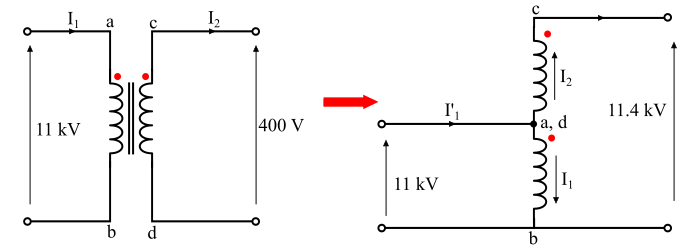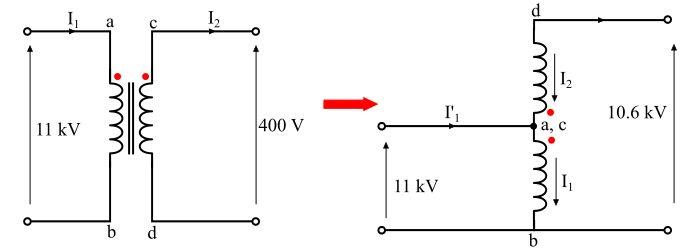# Conversion of a Two Winding Transformer into an Autotransformer

A conventional two-winding transformer can be converted into an autotransformer as shown in the figures given below. It can be converted into a step-up autotransformer by connecting the two windings electrically in series with additive polarities. If the windings are connected electrically in series with subtractive polarities, then a step-down autotransformer is obtained.

To understand the conversion of a two winding transformer into an autotransformer, consider a conventional two-winding transformer of 20 kVA, 11000/400 V. This transformer has to be connected in autotransformer.

## Case 1 – With Additive PolarityThe figure shows the series connections of the winding with additive polarity. As the polarity of the connection is additive, therefore, the output voltage on the secondary side is given by,

$$\mathrm{v_{2}=11000 + 400 = 11400\:V = 11.4 \:kV}$$

The primary side voltage is given by,

$$\mathrm{v_{1}= 11000\:V = 11\:kV}$$

Also, the current drawn by the autotransformer is given by,

$$\mathrm{{I^{'}}_{1}=I_{1}+I_{2}}$$

Hence, in this case, the transformer acts as a step-up autotransformer.

## Case 2 – With Subtractive Polarity

The connection diagram of this arrangement is shown in the figure. Here, the two windings are connected in series opposing so that it produces a reduced voltage across the secondary winding terminals. Hence, the transformer with subtractive polarity acts as a step-down autotransformer.For this case, the primary side and secondary side voltages are given as follows −

$$\mathrm{𝑉_{1} = 11000\:V = 11\:kV}$$

$$\mathrm{𝑉_{2} = 11000 − 400 = 10600\:V = 10.6 \:kV}$$

Also, the current drawn by the autotransformer is given by,

$$\mathrm{{I^{'}}_{1}=I_{1}+I_{2}}$$